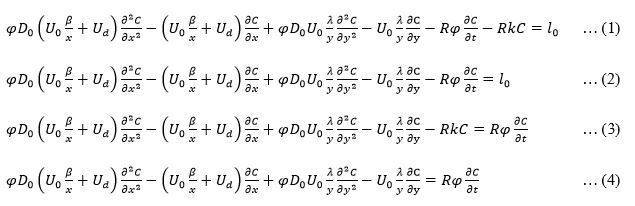# Partial Differntial Equation

• Nuel1647

## Homework Statement

The problem statement can be expressed in one of these forms listed in order of preference.[/B]

Every character with exception of x, y, t, and C are constants.

## Homework Equations

I require a change of variable or series of subsequent change of variables that can convert anyone of these equations into an equation having constant coefficients.

## The Attempt at a Solution

I have tried x1=(U0*beta + x*Ud)
I have also tried d/dx=((U0*beta/x)+Ud) d/dx1 and d/dy=y d/dy1

where x1 and y1 are the new variables for x and y respectively.
I know how to do the rest, like changing the boundary conditions and finding an a solution, I just need an appropriate equation for my variable transformation.
Any comment at all (including things like this equation is not solvable and why that is so) regarding the problem will be welcomed.

Is phi a function of x and y?

Chet